NEET  >  Magnet & Magnetic Field

# Magnet & Magnetic Field Notes | Study Physics Class 12 - NEET

## Document Description: Magnet & Magnetic Field for NEET 2022 is part of Physics Class 12 preparation. The notes and questions for Magnet & Magnetic Field have been prepared according to the NEET exam syllabus. Information about Magnet & Magnetic Field covers topics like and Magnet & Magnetic Field Example, for NEET 2022 Exam. Find important definitions, questions, notes, meanings, examples, exercises and tests below for Magnet & Magnetic Field.

Introduction of Magnet & Magnetic Field in English is available as part of our Physics Class 12 for NEET & Magnet & Magnetic Field in Hindi for Physics Class 12 course. Download more important topics related with notes, lectures and mock test series for NEET Exam by signing up for free. NEET: Magnet & Magnetic Field Notes | Study Physics Class 12 - NEET
 1 Crore+ students have signed up on EduRev. Have you?

16. Magnet:

16.1 Pole strength, magnetic dipole and magnetic dipole moment:

A magnet always has two poles `N' and `S' and like poles of two magnets repel other and the unlike poles of two magnets attract each other they form action reaction pair.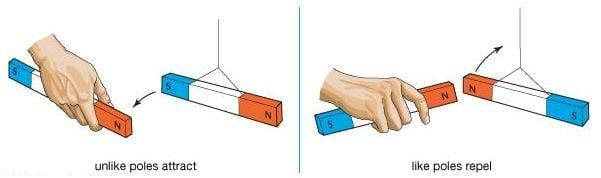Fig: Magnetic poles

The poles of the same magnet do not comes to meet each other due to attraction. They are maintained we cannot get two isolated poles by cutting the magnet from the middle. The other end becomes pole of opposite nature. SO, `N' and `S' always exist together.

Therefore, they are: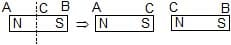Known as +ve and -ve poles. North pole is treated as positive pole (or positive magnetic charge) and the south pole is treated as -ve pole (or -ve magnetic charge). They are quantitatively represented by their "POLE STRENGTH" + m and - m respectively (just like we have charge +q and -q in electrostatics). Pole strength is a scalar quantity and represents the strength of the pole hence, of the magnet also).

A magnet can be treated as a dipole since it always has two opposite poles (just like in electric dipole we have two opposite charges -q and +q). It is called MAGNETIC DIPOLE and it has a direction is from -m to +m that means from `S' to `N').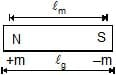M = m.lm here lm = magnetic length of the magnet. lm is slightly less than lg (it is geometrical length of the magnet = end to end distance). The `N' and `S' are not located exactly at the ends of the magnet. For calculation purposes we can assume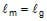[Actually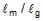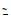0.84].

The units of m and M will be mentioned afterwards where you can remember and understand.

16.2 Magnetic field and strength of magnetic field:

The physical space around a magnetic pole has special influence due to which other pole experience a force. That special influence is called MAGNETIC FIELD and that force is called "MAGNETIC FORCE". This field is quantitatively represented by "STRENGTH OF MAGNETIC FIELD" or "MAGNETIC INDUCTION" or "MAGNETIC FLUX DENSITY". It is represented by . It is a vector quantity.

Definition of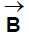: The magnetic force experienced by a north pole of unit pole strength at a point due to some other poles (called source) is called the strength of magnetic field at that point due to the source.

Mathematically,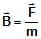Here = magnetic force on pole of pole strength m. m may be +ve or -ve and of any value. S.I. unit of is Tesla or Weber/m2 (abbreviated as T and Wb/m2).

We can also write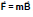. According to this direction of on +ve pole (North pole) will be in the direction of field and on -ve pole (south pole) it will be opposite to the direction of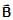.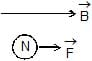and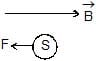The field generated by sources does not depend on the test pole (for its any value and any sign).
(A)due to various sources:

(i) Due to a single pole:

(Similar to the case of a point charge in electrostatics)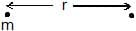B =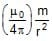.....(23)

This is magnitude

Direction of B due to north pole and due to south poles are as shown.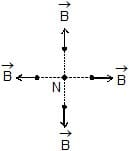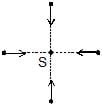in vector from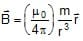...(24)

here m is with sign and = position vector of the test point with respect to the pole.

(ii) Due to a bar magnet:

(Same as the case of electric dipole in electrostatics) independent case never found. Always `N' and `S' exist together as magnet.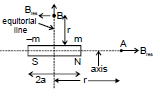at A (on the axis) = 2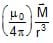for a << r ...(25)

at B (on the equatorial) = -for a << r ...(26)

At General point :

Br = 2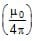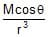Bn =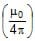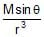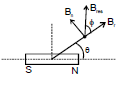Bres =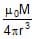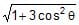...(27 (a))

tan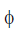=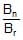=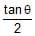...(28 (b))

Ex. 36: Find the magnetic force on a short magnet of magnetic dipole moment Mdue to another short magnet of magnetic dipole moment M1.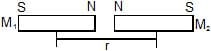Ans: To find the magnetic force we will use the formula of `B' due to a magnet. We will also assume m and -m as pole strengths of `N' and `S' of M2. Also length of M2 as 2a. B1 and B2 are the strengths of the magnetic field due to M1 at +m and -m respectively. They experience magnetic forces F1 and F2 as shown.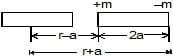F1 =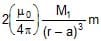and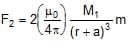Therefore, Fres F1-F2=2M1m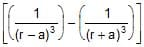=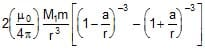By using, Binomial expansion, and neglecting terms of high power we get

Fres =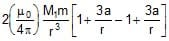=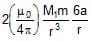=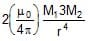=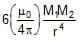Direction of Fres is towards right.

Alternative Method:

B =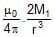⇒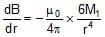F = - M2 ×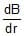⇒ F =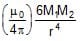Ex. 37:  Two short magnets A and B of magnetic dipole moments M1 and Mrespectively are placed as shown. The axis of `A' and the equatorial line of `B' are the same. Find the magnetic force on one magnet due to the other.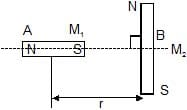Ans: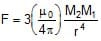upwards on M1

down wards on M2

Ex 38. A magnet is 10 cm long and its pole strength is 120 CGS units (1 CGS unit of pole strength = 0.1 A-m). Find the magnitude of the magnetic field B at a point on its axis at a distance 20 cm from it.

Ans: The pole strength is m = 120

CGS units = 12 A-m

Magnetic length is 2l = 10 cm or l = 0.05 m

Distance from the magnet is d = 20 cm = 0.2 m. The field B at a point in end-on position is

B =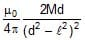=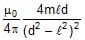=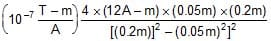=3.4×10-5 T.

Ex. 39: Find the magnetic field due to a dipole of magnetic moment 1.2 A-m2 at a point 1 m away from it in a direction making an angle of 60° with the dipole-axis.

Ans: The magnitude of the field is

B =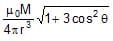=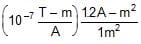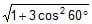The direction of the field makes an angle a with the radial line where

tan a ==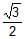Ex. 40: Figure shows two identical magnetic dipoles a and b of magnetic moments M each, placed at a separation d, with their axes perpendicular to each other. Find the magnetic field at the point P midway between the dipoles.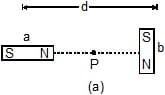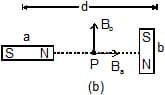Ans: The point p is in end-on position for the dipole a and in broadside-on position for the dipole b'. The magnetic field at P due to a is Ba =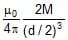along the axis of a, and that due to b is Bb =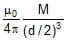parallel to the axis of b as shown in figure. The resultant field at P is, therefore

B =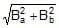=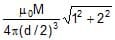=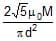The direction of this field makes an angle a with Ba such that tan a = Bb/Ba = 1/2.

16.3 Magnet in an external uniform magnetic field:

(same as case of electric dipole)

Fres = 0 (for any angle)

t = MB sin θ
* here θ is angle between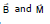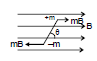Note: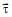acts such that it tries to make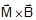is same about every point of the dipole it's potential energy is U = - MB cosθ = -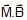θ= 0° is stable equilibrium

θ = p is unstable equilibrium

for small `q' the dipole performs SHM about q = 0° position

τ = - MB sin θ;

I α = - M B sin θ

α = -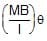for small θ, sin θθ

Angular frequency of SHM

ω=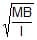=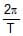⇒ T = 2p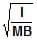here I = Icm if the dipole is free to rotate

= Ihinge if the dipole is hinged

Ex. 41: A bar magnet having a magnetic moment of 1.0 × 10-4 J/T is free to rotate in a horizontal plane. A horizontal magnetic field B = 4 × 10-5T exists in the space. Find the work done in rotating the magnet slowly from a direction parallel to the field to a direction 60° from the field.

Ans: The work done by the external agent = change in potential energy

= (-MB cosθ2) - (-MB cosθ1)

= - MB (cos60° - cos 0°)

=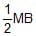=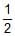× (1.0 × 104 J/T) (4 × 10-5 T) = 0.2 J

Ex. 42: A magnet of magnetic dipole moment M is released in a uniform magnetic field of induction B from the position shown in the figure.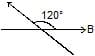Find:

(i) Its kinetic energy at θ = 90°

(ii) its maximum kinetic energy during the motion.

(iii) will it perform SHM? oscillation ? Periodic motion ? What is its amplitude ?

Ans: (i) Apply energy conservation at θ = 120° and θ = 90°

= - MB cos 120° + 0

= - MB cos 90° + (K.E)

KE =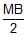Ans.

(ii) K.E. will be maximum where P.E. is minimum. P.E. is minimum at θ = 0º.

Now apply energy conservation between θ = 120º and θ = 0º.

= -mB cos 120º + 0

= - mB cos 0º + (KE)max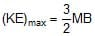Ans.

The K.E. is max at θ = 0° can also be proved by torque method. From θ = 120° to θ = 0° the torque always acts on the dipole in the same direction (here it is clockwise) so its K.E. keeps on increases till θ = 0°. Beyond that t reverses its direction and then K.E. starts decreasing. Therefore, θ = 0° is the orientation of M to here the maximum K.E.

(iii) Since `q' is not small.

Therefore, the motion is not S.H.M. but it is oscillatory and periodic amplitude is 120°.

Ex. 43: A bar magnet of mass 100 g, length 7.0 cm, width 1.0 cm and height 0.50 cm takes p/2 seconds to complete an oscillation in an oscillation magnetometer placed in a horizontal magnetic field of 25μT.

(a) Find the magnetic moment of the magnet.

(b) If the magnet is put in the magnetometer with its 0.50 cm edge horizontal, what would be the time period?

Ans: (a) The moment of inertia of the magnet about the axis of rotation is

I =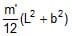=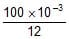[(7 × 10-2)2 + (1 × 10-2)2] kg-m2 =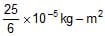We have, T =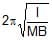or, M =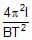=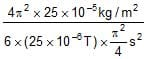= 27 A-m2

(b) In this case the moment of inertia becomes

I' =where b' = 0.5 cm.

The time period would be

T' =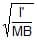...(ii)

Dividing by equation (i),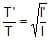=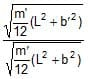=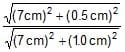= 0.992

or, T' =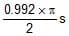= 0.496 p s.

16.4 Magnet in an External Non-uniform Magnetic field:

No special formula are applied is such problems. Instead see the force on individual poles and calculate the resultant force torque on the dipole.

Note:

• Force due to Non-uniform Magnetic field:

F =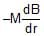• If a source of Magnetic Moment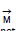have dimension very less than the distance of point of application then we can replace it with magnet of magnetic moment equal to.

17. TERRESTRIAL MAGNETISM:

Earth is a natural source of magnetic field.

17.1 Elements of the Earth's Magnetic Field:

The earth's magnetic field at a point on its surface is usually characterised by three quantities :

(a) declination

(b) inclination or dip and

(c) horizontal component of the field. These are known as the elements of the earth's magnetic field.

(a) Declination:

A plane passing through the geographical poles (that is, through the axis of rotation of the earth) and a given point P on the earth's surface is called the geographical meridian at the point P. Similarly, the plane passing through the geomagnetic poles (that is, through the dipole-axis of the earth) and the point P is called the magnetic meridian at the point P.

The angle made by the magnetic meridian at a point with the geographical meridian is called the declination at that point.

(b) Inclination or dip:

The angle made by the earth's magnetic field with the horizontal direction in the magnetic meridian, is called the inclination or dip at that point.

(c) Horizontal component of the earth's magnetic field:

As the name indicates, the horizontal component is component of the earth's magnetic field in the horizontal direction in the magnetic meridian. This direction is towards the magnetic north.

Figure shows the three elements. Starting from the geographical meridian we draw the magnetic meridian at an angle q (declination). In the magnetic meridian we draw the horizontal direction specifying magnetic north. The magnetic field is at an angle d (dip) from this direction. The horizontal component BH and the total field B are related as

BH = B cos δ

or, B = BH / cos δ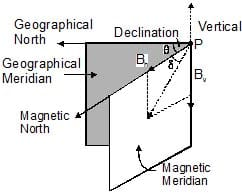Thus, from the knowledge of the three elements, both the magnitude and direction of the earth's magnetic field can be obtained.

Ex. 45 The horizontal component of the earth's magnetic field is 3.6 × 10-5 T where the dip is 60º. Find the magnitude of the earth's magnetic field.

Ans: We have BH = B cos δ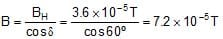The document Magnet & Magnetic Field Notes | Study Physics Class 12 - NEET is a part of the NEET Course Physics Class 12.
All you need of NEET at this link: NEET

## Physics Class 12

157 videos|452 docs|213 tests
 Use Code STAYHOME200 and get INR 200 additional OFF

## Physics Class 12

157 videos|452 docs|213 tests

### How to Prepare for NEET

Read our guide to prepare for NEET which is created by Toppers & the best Teachers

Track your progress, build streaks, highlight & save important lessons and more!

,

,

,

,

,

,

,

,

,

,

,

,

,

,

,

,

,

,

,

,

,

;